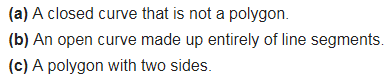# Illustrate, if possible, each one of the following with a rough diagram: (a) A closed curve that is not a polygon

Illustrate, if possible, each one of the following with a rough diagram: